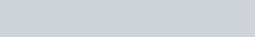In this video I introduce you to how we solve differential equations by separating the variables. I demonstrate the method by first talking you through differentiating a function by implicit differentiation and then show you how to solve

Solve:

1.by finding the general solution first and then use the initial conditions to find the particular solution.

Differential Equations (1) - Variables Separable : ExamSolutions - youtube Video

Which side does the Constant C go?

I am often asked which side should the constant of integration go. Well it doesn’t matter and hopefully this video will show you why.

Where does the Constant C go in a Differential Equation? : ExamSolutions Maths Revision - youtube Video